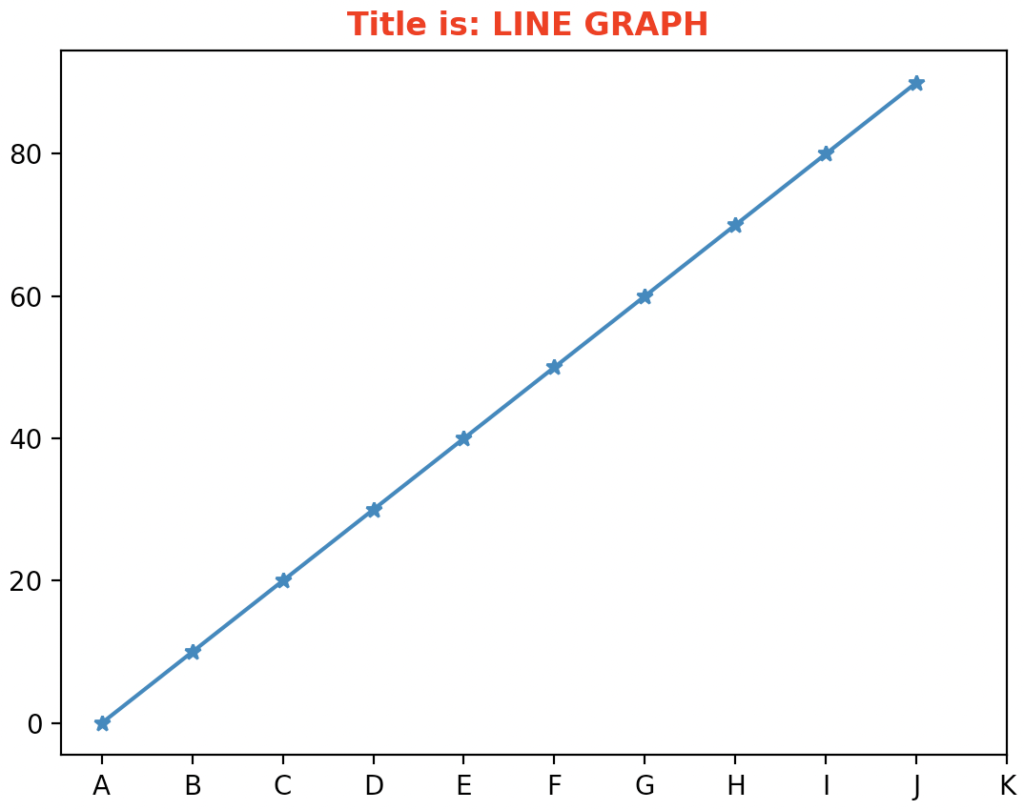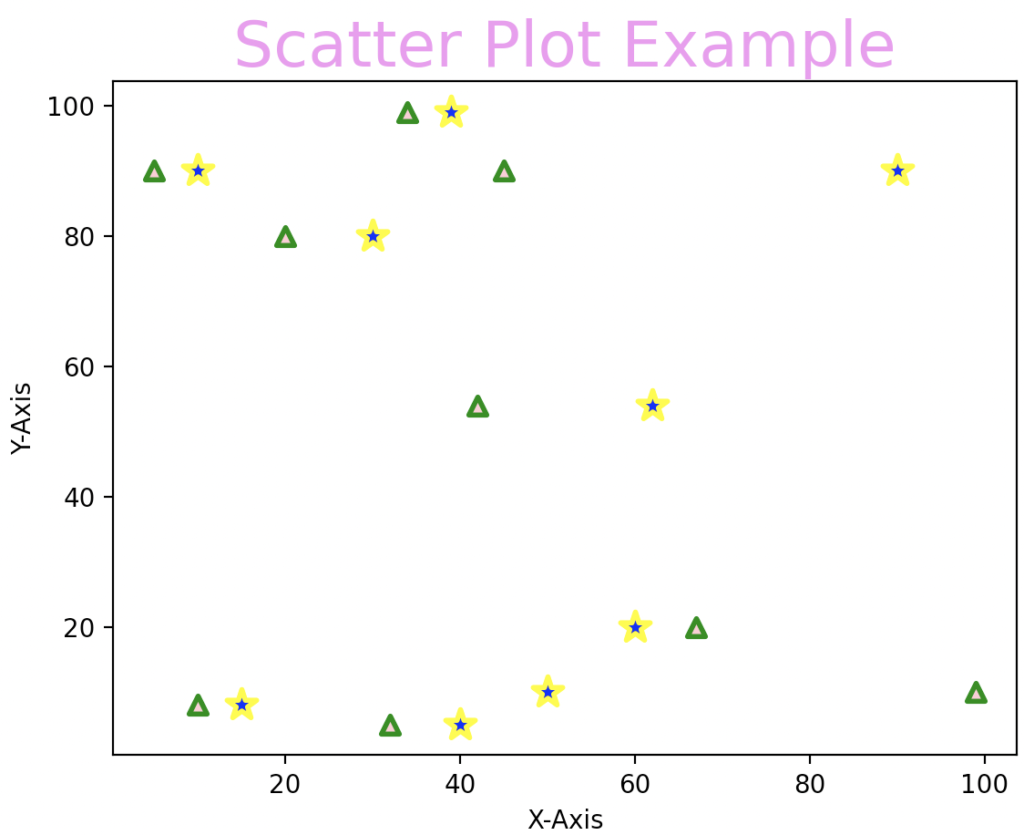# Matplotlib title: The Complete Guide

The title() function in the matplotlib library is used to specify the visualization title depicted and displays the title using various attributes.

## Matplotlib title

Matplotlib.pyplot.title() is a built-in library function that creates a title for the graph or chart. It adds the title to the created graph. It can be used for setting the title for the axes.

### Syntax

``matplotlib.pyplot.title(label, fontdict=None, loc=None, pad=None, *, y=None, **kwargs)``

### Parameters

The matplotlib.pyplot.title() function has one required argument as parameter:

1. label: This is the required argument. This argument takes values in string format. The text to be used as the title for the axe is passed in this argument. This text is kept as the title for the axes.
2. fontdict: The title text properties are passed in a dictionary for this argument. If nothing is passed, then the title’s default text property is used.
3. loc: This takes the location of the title as value. This argument accepts 3 values: center, left, and right. It describes the location where the title has to be kept. By default, it is kept as a center.
4. y: This takes floating point values as values. This describes the vertical axes location for the title.
5. pad: The offset of the title from the top of the axes is passed in this argument. This takes floating point values as value to this argument.

### Return value

The title() function returns the text instance as the value. The title() function returns the matplotlib text instance of the title as a return value.

### Program for creating a title for the line graph using matplotlib.pyplot.title

``````# Importing matplotlib.pyplot as plt.
import matplotlib.pyplot as plt

# Importing numpy as np
import numpy as np

# create a numpy array for storing the x coordinates
x = np.arange(0, 100, 10)

# create a numpy array for storing the y coordinates
y = np.arange(0, 100, 10)

# pass the x coordinates and y coordinates into the plot() function
plt.plot(x, y, '*-')

# Xticks is defined
plt.xticks([0, 10, 20, 30, 40, 50, 60, 70, 80, 90, 100], [
'A', 'B', 'C', 'D', 'E', 'F', 'G', 'H', 'I', 'J', 'K'])

# The title for the line graph is kept using the title function
plt.title("Title is: LINE GRAPH ", {'fontweight': 'bold', 'color': 'Red'})

# displaying the created graph using the show method
plt.show()``````

#### OutputIn this program, we imported matplotlib.pyplot for plotting the line chart and then for setting the title. The matplotlib library consists of all the functions for plotting different types of graphs and charts.

Then, we imported numpy for creating x coordinates. The x array consists of values from 0 to 100, with 10 as the interval. Then, we created the y array with the same interval.

The numpy array consists of x and y coordinates. Then, we plotted the line graph using the plot() function. We passed the format as * followed with – a line. Hence the points are marked with stars, connected with a line.

Then, we set the x-axis locations with the labels A to K. We passed the title for this plot using the title() function. We gave the font-weight as bold and then the color for the plot as red. Then we displayed the line chart using the show function. This displays the line graph with the title of the line graph as “Title is LINE GRAPH “.

### Program for creating a title for the scatter plot using matplotlib.pyplot.title

``````# Importing matplotlib.pyplot as plt.
import matplotlib.pyplot as plt

# Importing numpy as np
import numpy as np

# x coordinates are created
x = np.array([5, 20, 10, 67, 99, 45, 32, 34, 42])

# y coordinates are created
y = np.array([90, 80, 8, 20, 10, 90, 5, 99, 54])

# x1 coordinates are created
x1 = np.array([10, 30, 15, 60, 50, 90, 40, 39, 62])

# y1 coordinates are created
y1 = np.array([90, 80, 8, 20, 10, 90, 5, 99, 54])

# scatter plot is created
plt.scatter(x, y, c="pink",
linewidths=2,
marker="^",
edgecolor="green",
s=50)
plt.scatter(x1, y1, c="blue",
linewidths=2,
marker="*",
edgecolor="yellow",
s=150)

# x axis is labeled as X-Axis
plt.xlabel('X-Axis')

# y axis is labeled as Y-Axis
plt.ylabel('Y-Axis')

# Title is kept for the Scatter plot
plt.title('Scatter Plot Example', {"fontsize": 25, "color": "violet"})

# displaying the created graph using the show method
plt.show()``````

#### OutputIn this program, we imported matplotlib.pyplot to plot the scatter plot and set the title for the plot. A matplotlib library consists of all the functions for plotting different types of graphs and charts. Then we have imported numpy for creating x coordinates and y coordinates.

Then, we created another set of points called x1 and y1. Then, we passed x and y coordinates into the scatter function with the color pink, the edgecolor green, and the marker as a triangle.

Then, we passed the points x1 and y1 into the scatter function with the color blue, the edge color yellow, and the marker as a star. The function creates a scatter plot by combining x and y coordinates. Finally, we have used the title function for setting the title for the scatter plot.

The title() function is passed with the fontsize and color property. Then, we used the show function to display the generated scatter plot. In this example, two coordinates are plotted in a single scatter plot. Finally, we plotted the title for the graph.

That’s it for this tutorial.

This site uses Akismet to reduce spam. Learn how your comment data is processed.